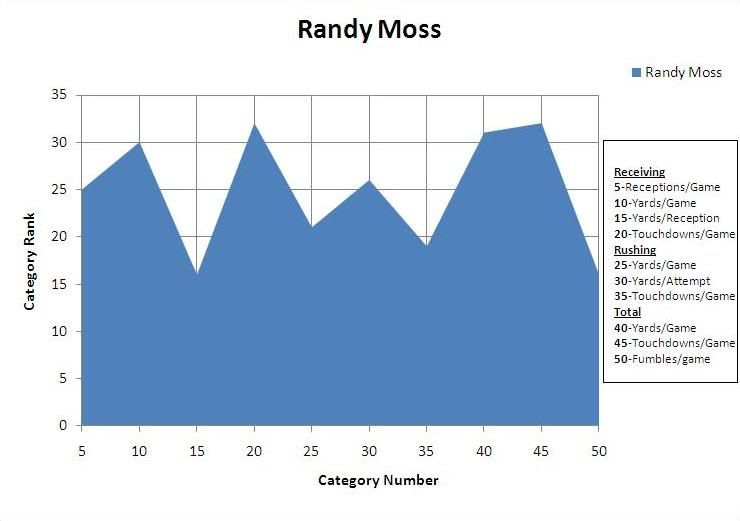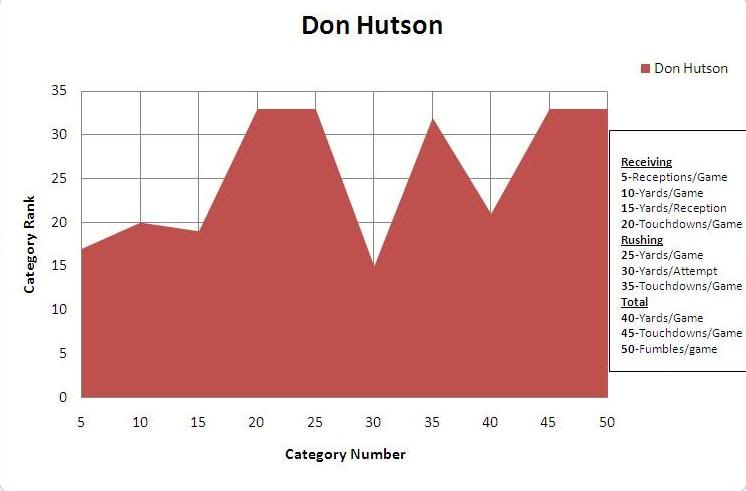X

# Is Randy Moss Greater Than Don Hutson?Who is greater?

Randy Moss/Don Hutson

Sports and STEM Project

Hyunsu Kong, Bleacher Report Contributor

Yanlan Dong

In this project we compared two of the top 33 greatest NFL wide receivers, Randy Moss and Don Hutson.

We compared the two using data from a study on Behind the Steel Curtain which ranked wide receivers from 1 to 33 in nine statistical categories such as “Receptions per Game.”

We used a mathematical modeling method. We found the total area under a multi-linear curve. Using the Fundamental Theorem of Calculus, we found the antiderivative of each linear section of the multi-linear curve.

From the data we compiled a graph for each player. By using the area under the curve for the players we could determine the greater of the two.

To do this we divided the graph into nine sections to represent the nine statistical categories. We determined the area of each section and then took the sum of those sections.

To find the slope we used the equation:

m=(y2-y1)/(x2-x1)

For Randy Moss the first two points are (5,25) and (10,30). So we plugged those two points into the equation:

(30-25)/(10-5)=5/5=>1

m=1

We then plugged the slope and the first ordered pair into the point-slope formula:

y-y1=m(x-x1)

y-25=1(x-5)

Then we rewrote the equation in slope-intercept form:

y=x+20

To get the area under the curve, we next took the integral of the equation:

∫510 (x+20)dx

[(½)x2+20x] 510

The quadratic expression is the antiderivative of

x+20.

((½)(10)2+20(10)) - ((½)(5)2+20(5))=137.5

The following is a list of the ordered pairs we used:

Don Hutson

(5,17) (10,20)

(10,20) (15,19)

(15,19) (20,33)

(20,33) (25,33)

(25,33) (30,15)

(30,15) (35,32)

(35,32) (40,21)

(40,21) (45,33)

(45,33) (50,33)

Randy Moss

(5,25) (10,30)

(10,30) (15,16)

(15,16) (20,32)

(20,32) (25,21)

(25,21) (30,26)

(30,26) (35,19)

(35,19) (40,31)

(40,31) (45,32)

(45,32) (50,16)

The total areas we calculated were:

Randy Moss=1137.5

Don Hutson=1155

This means Hutson is the greater of the two players.

Don Hutson is credited for creating many of the modern pass routes that current NFL players like Randy Moss use.

Statistical Data Source found here.